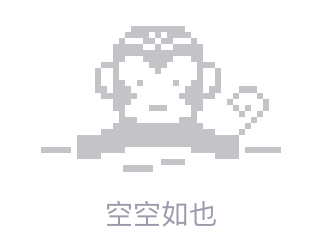# 从链接的textareas的\$ _POST数据中获取id

Trying to link two fields on a POST by getting the ID.

Each 'songfld' has a unique number as below. The textarea is linked on that number.

The form fields:

``````<input type="text" name="songfld" value="">
<textarea name="data[name]" id="txt-901" rows="2" cols="80"></textarea>

<input type="text" name="songfld" value="">
<textarea name="data[name]" id="txt-902" rows="2" cols="80"></textarea>
``````

And trying to get the 901 & 902 ID's here:

``````foreach(\$_POST['songfld'] as \$val){
\$val is the value from input
\$id = \$_POST['songfld'];     // the number in each name="songfld[X]"
\$namefld = \$_POST['data'][\$id]['name']; // gives the value from textarea

echo "<P>\$id \$idfld \$namefld";
}
``````

Of course the value passes, but I'd like to have that unique number from each post as well.

hope someone knows this trick.2019/06/25 13:12
• post
• php
• 点赞
• 收藏
• 回答
私信

#### 3个回复